# Advanced VLOOKUP in Excel: multiple, double, nested

These examples will teach you how to Vlookup multiple criteria, return a specific instance or all matches, do dynamic Vlookup in multiple sheets, and more.

It is the second part of the series that will help you harness the power of Excel VLOOKUP. The examples imply that you know how this function works. If not, it stands to reason to start with the basic uses of VLOOKUP in Excel.

Before moving further, let me briefly remind you the syntax:

VLOOKUP(lookup_value, table_array, col_index_num, [range_lookup])

Now that everyone is on the same page, let's take a closer look at the advanced VLOOKUP formula examples:

## How to Vlookup multiple criteria

The Excel VLOOKUP function is really helpful when it comes to searching across a database for a certain value. However, it lacks an important feature - its syntax allows for just one lookup value. But what if you want to look up with several conditions? There are a few different solutions for you to choose from.

### Formula 1. VLOOKUP with two criteria

Suppose you have a list of orders and want to find the quantity based on 2 criteria, Customer name and Product. A complicating factor is that each customer ordered multiple products, as shown in the table below: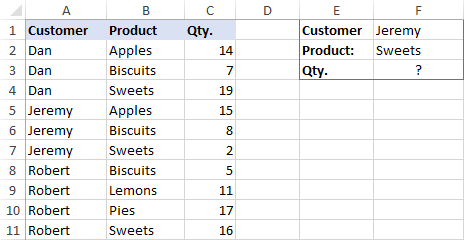A usual VLOOKUP formula won't work in this situation because it returns the first found match based on a single lookup value that you specify.

To overcome this, you can add a helper column and concatenate the values from two lookup columns (Customer and Product) there. It is important that the helper column should be the leftmost column in the table array because it's where Excel VLOOKUP always searches for the lookup value.

So, add a column to the left of your table and copy the below formula across that column. This will populate the helper column with the values from columns B and C (the space character is concatenated in between for better readability):

`=B2&" "&C2`

And then, use a standard VLOOKUP formula and place both criteria in the lookup_value argument, separated with a space:

`=VLOOKUP("Jeremy Sweets", A2:D11, 4, FALSE)`

Or, input the criteria in separate cells (G1 and G2 in our case) and concatenate those cells:

`=VLOOKUP(G1&" "&G2, A2:D11, 4, FALSE)`

As we want to return a value from column D, which is fourth in the table array, we use 4 for col_index_num. The range_lookup argument is set to FALSE to Vlookup an exact match. The screenshot below shows the result: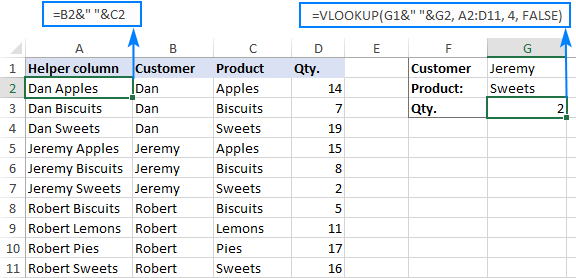In case your lookup table is in another sheet, include the sheet's name in your VLOOKUP formula. For example:

`=VLOOKUP(G1&" "&G2, Orders!A2:D11, 4, FALSE)`

Alternatively, create a named range for the lookup table (say, Orders) to make the formula easier-to-read:

`=VLOOKUP(G1&" "&G2, Orders, 4, FALSE)`

Note. For the formula to work correctly, the values in the helper column should be concatenated exactly the same way as in the lookup_value argument. For example, we used a space character to separate the criteria in both the helper column (B2&" "&C2) and VLOOKUP formula (G1&" "&G2).

### Formula 2. Excel VLOOKUP with multiple conditions

In theory, you can use the above approach to Vlookup more than two criteria. However, there are a couple of caveats. Firstly, a lookup value is limited to 255 characters, and secondly, the worksheet's design may not allow adding a helper column.

Luckily, Microsoft Excel often provides more than one way to do the same thing. To Vlookup multiple criteria, you can use either an INDEX MATCH combination or the XLOOKUP function recently introduced in Office 365.

For example, to look up based on 3 different values (Date, Customer name and Product), use one of the following formulas:

`=INDEX(D2:D11, MATCH(1, (G1=A2:A11) * (G2=B2:B11) * (G3=C2:C11), 0))`

`=XLOOKUP(1, (G1=A2:A11) * (G2=B2:B11) * (G3=C2:C11), D2:D11)`

Where:

• G1 is criteria 1 (date)
• G2 is criteria 2 (customer name)
• G3 is criteria 3 (product)
• A2:A11 is lookup range 1 (dates)
• B2:B11 is lookup range 2 (customer names)
• C2:C11 is lookup range 3 (products)
• D2:D11 is the return range (quantity)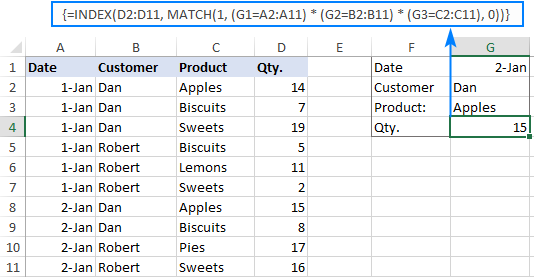Note. In all versions except Excel 365, INDEX MATCH should be entered as an CSE array formula by pressing Ctrl + Shift + Enter. In Excel 365 that supports dynamic arrays it also works as a regular formula.

For the detailed explanation of the formulas, please see:

## How to use VLOOKUP to get 2nd, 3rd or nth match

As you already know, Excel VLOOKUP can fetch only one matching value, more precisely, it returns the first found match. But what if there are several matches in your lookup array and you want to get the 2nd or 3rd instance? The task sounds quite intricate, but the solution does exist!

### Formula 1. Vlookup Nth instance

Suppose you have customer names in one column, the products they purchased in another, and you are looking to find the 2nd or 3rd product bought by a given customer.

The simplest way is to add a helper column to the left of the table like we did in the first example. But this time, we will populate it with customer names and occurrence numbers like "John Doe1", "John Doe2", etc.

To get the occurrence, use the COUNTIF function with a mixed range reference (the first reference is absolute and the second is relative like \$B\$2:B2). Since the relative reference changes based on a position of the cell where the formula is copied, in row 3 it will become \$B\$2:B3, in row 4 - \$B\$2:B4, and so on.

Concatenated with the customer name (B2), the formula takes this form:

`=B2&COUNTIF(\$B\$2:B2, B2)`

The above formula goes to A2, and then you copy it down to as many cells as needed.

After that, input the target name and occurrence number in separate cells (F1 and F2), and use the below formula to Vlookup a specific occurrence:

`=VLOOKUP(F1&F2, A2:C11, 3, FALSE)`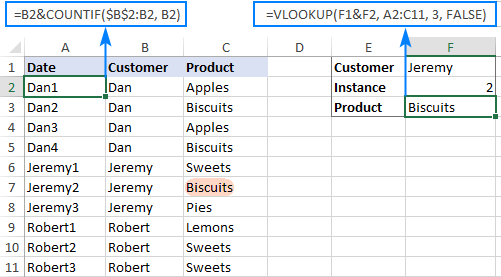### Formula 2. Vlookup 2nd occurrence

If you are looking for the 2nd instance of the lookup value, then you can do without the helper column. Instead, create the table array dynamically by using the INDIRECT function together with MATCH:

`=VLOOKUP(E1, INDIRECT("A"&(MATCH(E1, A2:A11, 0)+2)&":B11"), 2, FALSE)`

Where:

• E1 is the lookup value
• A2:A11 is the lookup range
• B11 is the last (bottom-right) cell of the lookup table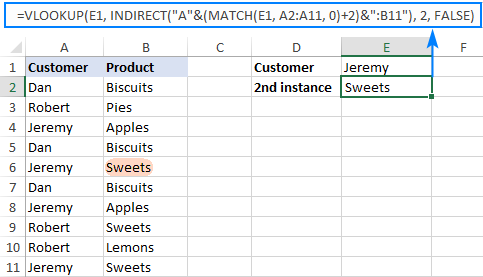Please note that the above formula is written for a specific case where data cells in the lookup table begin in row 2. If your table is somewhere in the middle of the sheet, use this universal formula, where A1 is the top-left cell of the lookup table containing a column header:

`=VLOOKUP(E1, INDIRECT("A"&(MATCH(E1, A2:A11, 0)+1+ROW(A1))&":B11"), 2, FALSE)`

#### How this formula works

Here is the key part of the formula that creates a dynamic vlookup range:

`INDIRECT("A"&(MATCH(E1, A2:A11, 0)+2)&":B11")`

The MATCH function configured for exact match (0 in the last argument) compares the target name (E1) against the list of names (A2:A11) and returns the position of the first found match, which is 3 in our case. This number is going to be used as the starting row coordinate for the vlookup range, so we add 2 to it (+1 to exclude the first instance and +1 to exclude row 1 with the column headers). Alternatively, you can use 1+ROW(A1) to calculate the necessary adjustment automatically based on the position of the header row (A1 in our case).

As the result, we get the following text string, which INDIRECT converts to a range reference:

`INDIRECT("A"&5&":B11") -> A5:B11`

This range goes to the table_array argument of VLOOKUP forcing it to start searching in row 5, leaving out the first instance of the lookup value:

`VLOOKUP(E1, A5:B11, 2, FALSE)`

## How to Vlookup and return multiple values in Excel

The Excel VLOOKUP function is designed to return just one match. Is there a way to Vlookup multiple instances? Yes, there is, though not an easy one. This requires a combined use of several functions such as INDEX, SMALL and ROW is an array formula.

For example, the below can find all occurrences of the lookup value F2 in the lookup range B2:B16 and return multiple matches from column C:

`{=IFERROR(INDEX(\$C\$2:\$C\$11, SMALL(IF(\$F\$1=\$B\$2:\$B\$11, ROW(\$C\$2:\$C\$11)-1,""), ROW()-1)),"")}`

There are 2 ways to enter the formula in your worksheet:

1. Type the formula in the first cell, press Ctrl + Shift + Enter, and then drag it down to a few more cells.
2. Select several adjacent cells in a single column (F1:F11 in the screenshot below), type the formula and press Ctrl + Shift + Enter to complete it.

Either way, the number of cells in which you enter the formula should be equal to or larger than the maximum number of possible matches.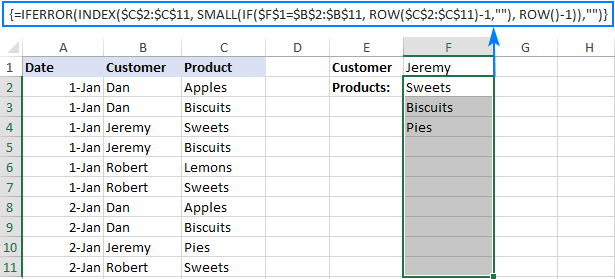For the detailed explanation of the formula logic and more examples, please see How to VLOOKUP multiple values in Excel.

## How to Vlookup in rows and columns (two-way lookup)

Two-way lookup (aka matrix lookup or 2-dimentional lookup) is a fancy word for looking up a value at the intersection of a certain row and column. There are a few different ways to do two-dimensional lookup in Excel, but since the focus of this tutorial is on the VLOOKUP function, we will naturally use it.

For this example, we'll take the below table with monthly sales and work out a VLOOKUP formula to retrieve the sales figure for a specific item in a given month.

With item names in A2:A9, month names in B1:F1, the target item in I1 and the target month in I2, the formula goes as follows:

`=VLOOKUP(I1, A2:F9, MATCH(I2, A1:F1, 0), FALSE)`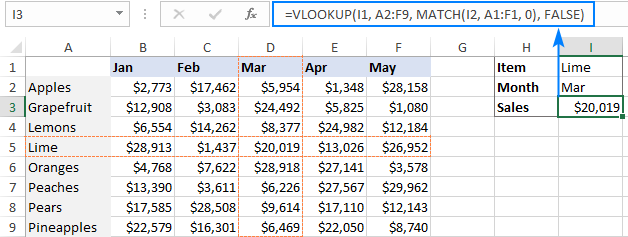### How this formula works

The core of the formula is the standard VLOOKUP function that searches for an exact match to the lookup value in I1. But since we do not know in which exactly column the sales for a specific month are, we cannot supply the column number directly to the col_index_num argument. To find that column, we use the following MATCH function:

`MATCH(I2, A1:F1, 0)`

Translated into English, the formula says: look up the I2 value in A1:F1 and return its relative position in the array. By supplying 0 to the 3rd argument, you instruct MATCH to find the value exactly equal to the lookup value (it's like using FALSE for the range_lookup argument of VLOOKUP).

Since Mar is in the 4th column in the lookup array, the MATCH function returns 4, which goes directly to the col_index_num argument of VLOOKUP:

`VLOOKUP(I1, A2:F9, 4, FALSE)`

Please pay attention that although the month names start in column B, we use A1:I1 for the lookup array. This is done in order for the number returned by MATCH to correspond to the column's position in table_array of VLOOKUP.

To learn more ways to perform matrix lookup in Excel, please see INDEX MATCH MATCH and other formulas for 2-dimensional lookup.

## How to do multiple Vlookup in Excel (nested Vlookup)

Sometimes it may happen that your main table and lookup table do not have a single column in common, which prevents you from doing a Vlookup between two tables. However, there exists another table, which does not contain the information you are looking for but has one common column with the main table and another common column with the lookup table.

In below image illustrates the situation: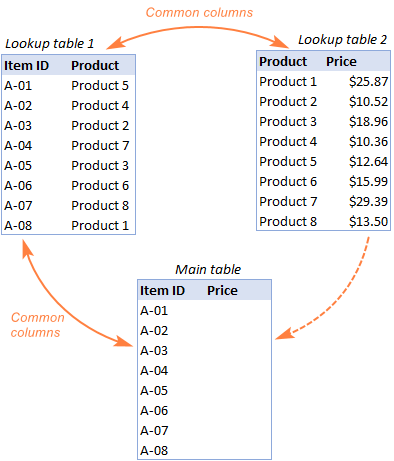The goal is to copy prices to the main table based on Item IDs. The problem is that the table containing prices does not have the Item IDs, meaning we will have to do two Vlookups in one formula.

For the sake of convenience, let's create a couple of named ranges first:

• Lookup table 1 is named Products (D3:E10)
• Lookup table 2 is named Prices (G3:H10)

The tables can be in the same or different worksheets.

And now, we will perform the so-called double Vlookup, aka nested Vlookup.

First, make a VLOOKUP formula to find the product name in the Lookup table 1 (named Products) based on the item id (A3):

`=VLOOKUP(A3, Products, 2, FALSE)`

Next, put the above formula in the lookup_value argument of another VLOOKUP function to pull prices from Lookup table 2 (named Prices) based on the product name returned by the nested VLOOKUP:

`=VLOOKUP(VLOOKUP(A3, Products, 2, FALSE), Prices, 2, FALSE)`

The screenshot below shows our nested Vlookup formula in action: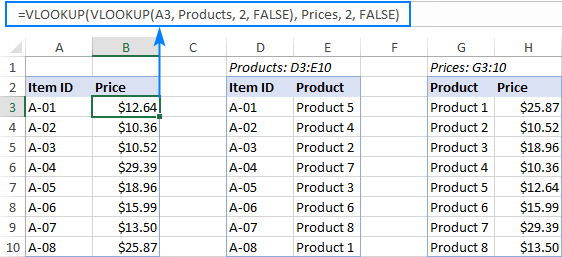## How to Vlookup multiple sheets dynamically

Sometimes, you may have data in the same format split over several worksheets. And your aim is to pull data from a specific sheet depending on the key value in a given cell.

This may be easier to understand from an example. Let's say, you have a few regional sales reports in the same format, and you are looking to get the sales figures for a specific product in certain regions: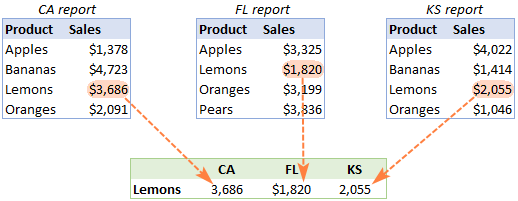Like in the previous example, we start with defining a few names:

• Range A2:B5 in CA sheet is named CA_Sales.
• Range A2:B5 in FL sheet is named FL_Sales.
• Range A2:B5 in KS sheet is named KS_Sales.

As you can see, all the named ranges have a common part (Sales) and unique parts (CA, FL, KS). Please be sure to name your ranges in a similar manner as it's essential for the formula we are going to build.

### Formula 1. INDIRECT VLOOKUP to dynamically pull data from different sheets

If your task is to retrieve data from multiple sheets, a VLOOKUP INDIRECT formula is the best solution – compact and easy-to-understand.

For this example, we organize the summary table in this way:

• Input the products of interest in A2 and A3. Those are our lookup values.
• Enter the unique parts of the named ranges in B1, C1 and D1.

And now, we concatenate the cell containing the unique part (B1) with the common part ("_Sales"), and feed the resulting string to INDIRECT:

`INDIRECT(B\$1&"_Sales")`

The INDIRECT function transforms the string into a name that Excel can understand, and you put it in the table_array argument of VLOOKUP:

`=VLOOKUP(\$A2, INDIRECT(B\$1&"_Sales"), 2, FALSE)`

The above formula goes to B2, and then you copy it down and to the right.

Please pay attention that, in the lookup value (\$A2), we've locked the column coordinate with absolute cell reference so that the column remains fixed when the formula is copied to the right. In the B\$1 reference, we locked the row because we want the column coordinate to change and supply an appropriate name part to INDIRECT depending on the column into which the formula is copied: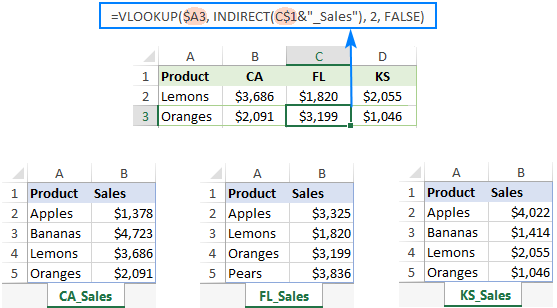If your main table is organized differently, the lookup values in a row and unique parts of the range names in a column, then you should lock the row coordinate in the lookup value (B\$1) and the column coordinate in the name parts (\$A2):

`=VLOOKUP(B\$1, INDIRECT(\$A2&"_Sales"), 2, FALSE)`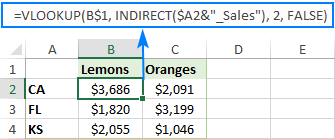### Formula 2. VLOOKUP and nested IFs to look up multiple sheets

In situation when you have just two or three lookup sheets, you can use a fairly simple VLOOKUP formula with nested IF functions to select the correct sheet based on the key value in a particular cell:

`=VLOOKUP(\$A2, IF(B\$1="CA", CA_Sales, IF(B\$1="FL", FL_Sales, IF(B\$1="KS", KS_Sales,""))), 2, FALSE)`

Where \$A2 is the lookup value (item name) and B\$1 is the key value (state):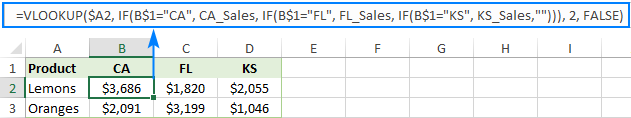In this case, you do not necessarily need to define names and can use external references to refer to another sheet or workbook.

For more formula examples, please see How to VLOOKUP across multiple sheets in Excel.

That's how to use VLOOKUP in Excel. I thank you for reading and hope to see you on our blog next week!

Advanced VLOOKUP formula examples (.xlsx file)

## You may also be interested in

=IFERROR(INDEX(E5:E11,SMALL(IF(E2=E5:E11,ROW(F5:F11)-1,""),ROW()-3)),"")

• Lineth,

Please make sure you press Ctrl + Shift + Enter to enter the array formula correctly.

2. I am quite poor on excel and can do simple VLookups. I need to do one that I think should in corporate an If function but do not know how. I need it to Vlookup a selection of codes and return the rate from column 2. However for 1 code I need it to go to one list for the answer, for all other codes I need it to go to another list for the answer. e.g I have 10 carrier codes 9 of the codes have a fixed rate - easy but one of the codes has a different rate for every item. So for the 9 with a fixed rate easy however how do I tell it that if code is this odd one go lookup on this list but if not go lookup on that list? I have played around for days trying to work it out. hope that this makes sense

3. Hi Alireza,
for post 54. you can use the following array formula without sorting your first column:
=max(if(b2:b15=e2,c2:c15))
use CSE.
Good luck.

4. Dear all am looking for some help

I have a cell with code in it and than a seperated sheet whit the same codes and some devided lanes exp
cell M17 = TYO
in sheet 2 I have a range A24 untill B 39 were TYO is mentioned 4 times in A and the in B there is TYO+ each time a different explanation

now I would like to find a formula to place in sheet one M17 is TYO that the various explanations apeare

5. Hi - I have a table with 3 columns: Course Name, Credits and Category
IE
100W 3 GE
117a 4 GE
17a 3 Comp
56 3 Surveys

I have a worksheet with the following columns: Instructor, Course Name, Total Credits

Cindy Miller 17a 3
Shannon Baer 17a 3
Bill Jones 56 3

I created a lookup table to calculate the total credits based on the selected course name and looking at the credits associated with same in the table.

This works fine.

Now I want to determine the quantity of courses for each Category.

So in the example of Cindy, Shannon and Bill it would look like this:

Category # of Courses
Comp 2 (since course 17a is a category Comp and Shannon and Cindy are teaching)
Surveys 1 (since course 56 is a category Survey and Bill is teaching)

Can't wrap my head around how to get the count of courses offered by category.

My query is that how I can use ">" & "<" greater than & Less than in single formula in excel sheet by solving the problem.
QN.:Find the value greater than 250 and Less than 800.
Example:

Name Amount Age
Bill 256.95 56
Joe 125.63 22
Mary 25.66 59
Dave 548.00 21
Frank 489.32 48
Sue 500.25 19
Hillary 368.59 15
Kate 901.56 25
Aleisha \$99.95 33

7. I have two spresdsheets - one having a customer name in column A and product name in column d and another spreadsheet having net price for the same criteria. The net price are different for same product for diffferent customers. I am trying to bring the net price to the first spreadsheet. What is the formula to use? Thanks

8. Great help to me as a novice. Is there anyway you could assist with the following problem;
In a cell I have a date which can be changed eg, Aug 2015, Sep 2015, Oct 2015 etc. This in essence represents the sheet name containing the main look up table.Is there a variant of the the vlookup formula below that:
Updates the formula with the date (sheet tab name) based on the selection in the date cell?
EXAMPLE: If Sep 2015 selected in date cell, then return the formula ,=VLOOKUP(A6,'SEP 2015'!\$B\$5:\$L\$287,7,FALSE).
If Oct 2015 selected in date cell, then return the formula,=VLOOKUP(A6,'OCT 2015'!\$B\$5:\$L\$287,7,FALSE).
Any help would be greatly appreciated thanks!

9. I have two tables Main table and Vlookup table. Vlookup table as "place_table". Vlookup table has three sheets of seperated places sheet1 as Mumbai, sheet2 as Delhi, sheet3 as Chandigarh.In main table I am using vlookup formulas seperately =vlookup(b2 ,[place_table.xlsx]Mumabai!1:1048576,2,0), =vlookup(b2 ,[place_table.xlsx]Delhi!1:1048576,2,0), =vlookup(b2 ,[place_table.xlsx]Chandigarh!1:1048576,2,0). In main table I have seperate columb which shows places Mumbai, Delhi and Chandigarh. How can I use this columb to applying only one formulas to all

for one formula for all three sheets.

10. Hi again:)

After a night thinking about the problem above I have answered my own question.

To increment the "-20" I placed the following in an unused cell on row 20, (in my case this cell is in a column that is normally hidden).

"=row()"

this shows the row number & updates every time a row is added above it.

I named this cell "row_increment"

Then I adjusted the code above:-
),ROW()-20)),"Error")}

),ROW()-row_increment)),"Error")}

So every time I add a new row ABOVE row 20 [=row()] automatically updated the -20 to -21, -22 etc
My table no longer looses the top row

I hope this makes sense to others.

SteveN

11. Hi

I've copied and modified your example above for finding duplicate values.
my code:
'{=IFERROR(INDEX(expedite_report!\$H\$8:\$H\$32000,SMALL(IF(\$B\$21=expedite_report!\$A:\$A,ROW(expedite_report!\$H\$8:\$H\$32000)-14,""),ROW()-20)),"Error")}

This works very well thank you :)

I have an issue tho'
The worksheet is growing all the time with new rows being added which then impacts "ROW()-20". The \$B\$21 increments as new rows are added but the "-20" does not & I'm loosing results off the top of the array.

How can I modify this code such that the "-20" increments when a new row or rows are added?

SteveN

12. I am trying to create a forumala which will allow me to do the following:

eg. if the figure in A2 IS the greatest column A then it is 10 and if its 2nd Greatest it is 9 etc.

13. If you send me some advanced excel formulas, I will be greatful....

• Hi SUBHAM,

14. Hi, i have a table with thee sheets with numerous addresses on each sheet. I want to search each of the three sheets and only return the value if the address appears on all three sheets. How do I do this?

15. This is wonderful, I have found this and one other article very helpful. One question, if you have a moment, can you refer me to an article similar to this but where the second look-up term is actually a range? For Names: John, Fred, Mark and Number of Items are either 0-10, 11-20, 21and greater.

So basically, one would select John, and enter a number, say 19, and the returning would would be Red or if one A1=Fred, B1=25 - looking for a formula that would return the result of Warm - based on the example table below.
Thanks!
0-10 | 11-20 | 21 and up
John Bronze |Red | Hot
Fred Silver |Orange | Warm
Mark Gold |Green | Cole

16. Hello

I would like to use the formula "How to do two-way lookup in Excel" and instead of getting the value at the intersection i would like to just paint or fill the cell with a color.

Any help would be appreciated.
Ralph

A B C
item product cost
9501 orange 3.5
9502 apple 4.5
9503 pear 6.0

18. How can I combine two VLookup formulas together, for example the formula I am using is =if(A1=","",VLOOKUP(A1,PRODUCTS,3,FALSE)&IF(A1=","",VLOOKUP(A1,PRODUCTS,2,FALSE))) in return I am getting two answers when I only want one answer not both, if I enter criteria 3 I want to get criteria 3 and if I enter criteria 2 I want to get criteria 2 not both at the same time like I am getting now.
item product cost
9501

19. Hi,

Thank you for your very useful posts and I hope you can help with a problem I have:

I have a cell (C6) which contains text based on a vlookup formula. I want another cell (G6) to return either "True" or "False" based on the text in C6. In G6 I have 'IF(C6="Air Cooled","True","False") but this doesn't work. If I just type "Air Cooled" into C6 then it's fine, G6 returns "True".

Can you please tell me if there is a way around this?

Thank you.

• Hi Anne,

Your formula is correct and it should work both for values typed manually and returned by other formulas, and it works just fine in my test sheet. An immediate reason for the formula not working that comes to mind is that the value returned by your Vlookup formula has some slight difference in spelling, or a double space between words, leading or trailing space, etc.

20. Hi, I was wondering if you could help me please?

I am trying to do a two way VLOOKUP , I have sales invoice numbers and purchase invoice numbers as the initial look up cells, I then want to search for these invoices in two other worksheets, I then would like to column next to the invoice number on the other sheets to appear. I have this at the moment but I does not appear to work,

=IF(ISNA(VLOOKUP(D39,'Purchase Ledger Control'!B13:B33,2,0)),"",IF(VLOOKUP(D39,'Purchase Ledger Control'!B13:B33,2,0)="",""&IF(ISNA(VLOOKUP(E39,'Sales Ledger Control'!B13:B30,2,0)),"",IF(VLOOKUP(E39,'Sales Ledger Control'!B13:B30,2,0)="",""))))

Chris

21. how i should proceed for more than two cloumn lookup criteria.

22. I want 0 inspite of #N/A when ever vlookup comes up with no value (& there is also actually not any value against that respective reference).Can you kindly tell me how can I get that. I used If(iserror also but it brings "Blank" cell when there is #N/A but our requirement is 0.

Help is greatly appreciated

You can use the IFNA function with 0 in the second argument, like this:
=IFNA(VLOOKUP(), 0)

If you want the formula to bring 0 instead of any errors, not only #N/A, then use the IFERROR function:
=IFERROR(VLOOKUP(), 0)

23. Hello,
Can you explain how to get all Duplicate values in a lookup range, looking up values in a different worksheet(of the same workbook)?

24. Hi!

If i want the multiple results to be displayed in columns how will i do it with this?

{=IFERROR(INDEX(\$C\$2:\$C\$16,SMALL(IF(\$F\$2=B2:B16,ROW(C2:C16)-1,""),ROW()-3)),"")}

Thank you!

25. Thanks for the great post. Nevertheless, I have been presented a problem to interpolate in a given lookup "string" which is concatenated a "value" . As an example :

The string here would represent the pipe name, value is the pipe number, value2 represents the depth and value 3 is the x-coordinate.
--String Value Value2 Value 3
Pipe 1 200 50
Pipe 1 210 60
Pipe 2 200 45
Pipe 2 250 60
Pipe 3 150 50
Pipe 3 200 55

I'd like to create a lookup function which I can not only identify but interpolate the x-coordinate (value 3) at a given pipe depth (value 2) and pipe identification (String and value) .

Naturally I have tried to use combined "helper" (=Pipe&3&150) but unfortunately excel treats this a string but not values. This can only work if I provide the exact depth which would return the exact coordinate value on the table.

Any advice, my respected excel gurus?

• Izwan,

For us to be able to assist you better, please send your sample workbook with the source data and expected result to support@ablebits.com. I am sorry, at the moment it is not very clear why you need interpolation.

26. I am trying to use Vlookup in pulling out the grades from a transmutation table. Column A is the lookup value and column B is the equivalent grade. The values in A begin with 0 and increments of 0.01 while column B begins with 60. When this formula is used: =VLOOKUP(T4,Sheet2!\$A\$1:\$B\$10000,2,FALSE) the returning value is #N/A.

27. Hi, I have been trying to figure this out for some time now so I hope you can help - I have 2 seperate spreadsheets that I need to combine into a sales forecast.

Basically, I have 2 tabs in my sales and stock forecast -

1st sheet has product SKUs in stock, and quantity
2nd sheet has product SKUs and sales in units, not £

I paste into the stock sheet from an external source, and also the sales from a different source - hence the seperation. The SKUs in stock may not always match the SKU sales, so I need to first match the SKUs, to save trolling through a large number of them, and then transfer over the sold units.

eg:

SKUs in stock
tshirt 2
bottle 4
shorts 2
cap 3

SKU sales
tshirt 1
bottle 2
cap 1

So, I think, I need to match tshirt, bottle and cap in the forecast sheet and then match over the sales for each so I can then forecast the next stock.

I hope this makes sense?!

28. Dear all, I want data in below given foramt kindly help me

Source Cell Neighour Cell Source Cell NB1 NB2 NB3 NB4
A 1 A 1 2 3 4
A 2 B 4 5 6 19
A 3 C 7 8 9 10
B 4
B 5
B 6
C 7
C 8
C 9
C 10

Thanks

29. I have 2 reports; one of previous report and one of current report.
In the current report, I run vlookup against the previous report for dates to identify new entries and old entries. After which, I run true false to identify whether the dates of the previous report match/differs with the dates of the current report.
The purpose is to check what are the new entries and also to check if there are date changes from the previous entries.
The challenge is I create two columns, one for vlookup, one for true false.
Is there anyway to combine both formulas and have just one column?

30. Hi, seek help on below.

Item1 1.05 A
Item1 2.50 B
Item1 7.85 C

I need a formula to match both below:
-item
-price that could different by +/-0.50

Example, if I lookup :
a. Item1, 2.00 - Return B
b. Item 1, 5.00 - Error

Would really appreciate any help or just throw in any ideas as brainstorm. Thanks

31. Team, i need code for below:

Input:

A 12
A 11
A 10
B 11
B 12

Out put:
A 12 11 10
B 11 12

32. Hi..super work and brilliant ideas. I have a problem in excel that I hope you may be able to help me with. I have a worksheet in which I want to find multiple values that correspond to a merge cell. My worksheet contains Buses 1-4 (A4:A11), each bus in 2 merged cells (for example Bus 1 in merged cells (A4:A5) and each bus has 2 routes (R1 & R2) and 3 stops (S1, S2, S3)in each route in the morning (AM), afternoon (PM), and night (HS). I want vlookup to find the times each bus in waits in each stop for each route at the three different periods (AM, PM, HS). Seems complex by I dried a hundred times to solve it..no way. Any suggestions? I can provide you with a screen snapshot of my excel sheet, or upload it if that helps. Thanks a million
Samer

33. Thanks
info helped me a lot

34. how many types of vlookup exist?

35. Sl.No Inv. Ref. No. Inv.Rcvd. Date Status Month Vendor Code Vendor Name Invoice Date Job Ref. ID Inv. Amount 7 % GST Total Inv. Value Due Date Credit Days (terms)
RE/PY/17 000017 8-Jan-13 PD 1-2013 0 EUROSAFE PTE LTD DECEMBER 3-Jan-15 R356 \$36.90 \$- \$36.90 #N/A #N/A
RE/PY/18 000018 24-Jan-13 PD 1-2013 0 VICTORIA PROPERTIES & INVESTMENT PTE LTD IN0000236125 23-Dec-14 R405 \$2,519.23 \$176.35 \$2,695.58 #N/A #N/A
RE/PY/19 000019 24-Jan-13 PD 1-2013 0 SP SERVICES LTD PSI-V9-77756 17-Dec-14 R405 \$3,132.00 \$219.24 \$3,351.24 #N/A #N/A
RE/PY/20 000020 24-Jan-13 PD 1-2013 0 SMS DESIGN PTE LTD PSI-V9-77755 20-Dec-14 R405 \$1,350.65 \$94.55 \$1,445.20 #N/A #N/A
RE/PY/21 000021 6-Feb-13 PD 2-2013 0 SWOT TECHNOLOGIES PVT. LTD. LSPI-001596 22-Dec-14 R405 \$1,400.00 \$98.00 \$1,498.00 #N/A #N/A
RE/PY/22 000022 7-Jun-13 PD 6-2013 0 EUROSAFE PTE LTD 4517 9-Dec-14 R400 \$530.00 \$37.10 \$567.10 #N/A #N/A
RE/PY/23 000023 20-Feb-13 PD 2-2013 0 SMS DESIGN PTE LTD INV-2014-074 22-Dec-14 R405 \$10,330.00 \$723.10 \$11,053.10 #N/A #N/A
RE/PY/24 000024 20-Feb-13 PD 2-2013 0 SMS DESIGN PTE LTD TU-IV1501-0119 1-Jan-15 R356 \$3,480.00 \$109.20 \$3,589.20 #N/A #N/A
RE/PY/25 000025 21-Feb-13 PD 2-2013 0 SMS CONSULTING ENGINEERS PROGRESS CLAIM NO.2 6-Nov-14 R400 \$12,554.25 \$878.80 \$13,433.05 #N/A #N/A
RE/PY/26 000026 25-Feb-13 PD 2-2013 0 VICTORIA PROPERTIES & INVESTMENT PTE LTD PT-2013-3512 20-Nov-13 R390 \$350.00 \$24.50 \$374.50 #N/A #N/A
RE/PY/27 000027 25-Feb-13 PD 2-2013 0 SP SERVICES LTD ADVANCE 7-Jan-15 R356 \$25,000.00 \$- \$25,000.00 #N/A #N/A
RE/PY/28 000028 12-Mar-13 PD 3-2013 0 EUROSAFE PTE LTD 11/348 24-May-11 R356 \$1,000.00 \$- \$1,000.00 #N/A #N/A
RE/PY/29 000029 12-Mar-13 PD 3-2013 0 SMS DESIGN PTE LTD 119618/2Q 31-Dec-14 R357 \$50.00 \$3.50 \$53.50 #N/A #N/A
RE/PY/30 000030 22-Mar-13 PD 3-2013 0 SP SERVICES LTD 76666 25-Jul-14 R357 \$33.00 \$- \$33.00 #N/A #N/A
RE/PY/31 000031 28-Mar-13 PD 3-2013 0 VICTORIA PROPERTIES & INVESTMENT PTE LTD PSI-V9-78007 27-Dec-14 R405 \$1,147.50 \$80.33 \$1,227.83 #N/A #N/A
RE/PY/32 000032 3-Apr-13 PD 4-2013 0 SP SERVICES LTD PSI-V9-78008 26-Dec-14 R405 \$3,272.50 \$229.08 \$3,501.58 #N/A #N/A
RE/PY/33 000033 3-Apr-13 PD 4-2013 0 SMS DESIGN PTE LTD 108020970 22-Dec-14 R405 \$5,232.00 \$366.24 \$5,598.24 #N/A #N/A

36. =VLOOKUP(G2,Table2[[Vendor Name]:[Vendor code2]],2,0) in need to some example this formulas i try to previous work based but i couldn't completed so kingly updated this

37. Hello Svetlana, I want to create a formula that allows me to firstly find a matching code, then to find a matching date then once both of those items are found to populate the cell with the figure (this figure would be in the cell below the date) see below for example.

Example 1 (I want to create the formula in the #REF cell under the 24,000):
SOH 24,000
Total OPENING SOH 24,000 #REF! #REF! #REF!
Demand #REF! #N/A #N/A #N/A
Projected Closing Stock #REF! #REF! #REF! #REF!

Example 2 (this data is on a separate worksheet within the same spreadsheet):

31/03/2015 1/04/2015 8/07/2014 19/08/2014
29820 Basket 21760 27200 27200 21760 59840 27200 27200

So ultimately I want to be able to firstly find the code 29820 in the worksheet, then if the date in the worksheet matches the same date in the main worksheet to populate that cell with the number below the date?

Is this at all possible?

Thanks,

38. How to assign same value to a cell using dependent list?
For(your)example: how can I assign/populate same list for two values(fruits) say 'Mango' and 'Apricot' dynamically without duplicating the list
values?

39. thank you for your detail explanation
but i'm working with price table for the same product has more than one price ( from different supplier) i would like to be the output is the lowest price below

pipe material pipe size pipe type pipe price pipe supplier
pvc 100 sch40 62\$ aapco
pvc 150 sch40 72\$ aapco
pvc 200 sch40 82\$ aapco
pvc 100 sch40 55\$ amis
so i need the out if the pipe material is pvc and size is 100 and type is sch40 the lowest price (55)?

40. Svetlana,

Thank you so much for this very helpful post. I'm trying to use the array formula for duplicate values in a range.

=INDEX('Cert II Unit Selector'!\$B\$30:\$B\$39,SMALL(IF('Cert II Unit Selector'!K30:K39="Y",ROW('Cert II Unit Selector'!B30:B39)-1,""),ROW()-3))

I keep getting a #Value error. Essentially, a "Y" is placed next to different selections out of many(say 16 out of 50), and i'd like the title of those labelled "Y" to be copied down row by row onto another worksheet.

Your formula seems to be the best option for this (I'm VBA shy) but I can't get it to work? Am I doing something wrong?

41. Hi Svetlana,
Your tutorials and instructions are fantastic! I am under the impression that the function/formula I am trying to create is not possible in Excel and I am at my wits end. Here is the basic gist:

Column A contains various vendors
Column B contains various account types

I would like to have it so that each cell in an entire sheet will provide the results of each criteria (all of the data is text)

Column A header is ingredient 1

Column A2: Eggs
Column B2: Bacon
therefore.....
Column C2: Delicious

Column A3: Yogurt
Column B3: Cheese
therefore.....
Column C3: Gross

Column A4: Banana
Column B4: Peanut Butter
therefore.....
Column C4: Healthy

I am happy to send over a sample spreadsheet I have that I've been playing with. The report I use typically has 200-300 rows of data. Currently this analysis is done manually and takes DAYS. Thank you!

42. Saved me hours of formula testing and frustration.
5 mins of reading saved hours of vlookup frustrations.

Thank you very much

43. this is relay very help full for me thank your dear Svetlana Cheusheva.

44. i have a worksheet with various data, i want to transfer some selected columns and multiple rows at the same time in other worksheet using vba

45. Hi,

I have a data of staff name, date and daily productivity.

Data catagory as "Actuals"
A B C D E
Date Name Productivity % Agent Occupancy AHT
05-Jan-15 Staff A 107 51.42 129
06-Jan-15 Staff A 77 49.58 167
07-Jan-15 Staff A 100 62.69 162
05-Jan-15 Staff B 68 61.99 198
06-Jan-15 Staff B 50 64.38 321
07-Jan-15 Staff B 67 74.51 255
05-Jan-15 Staff C 32 73.20 192
06-Jan-15 Staff C 20 59.11 194
07-Jan-15 Staff C 66 57.87 176

How can i lookup for Staff A, C=B & C Performance as below?

05-Jan-15 06-Jan-15 07-Jan-15

Productivity
Staff A =VLOOKUP("Staff A",Actuals,MATCH(05-Jan-15,Actuals,0),FALSE)
Staff B
Staff C

% Agent Occupancy
Staff A
Staff B
Staff C

AHT
Staff A
Staff B
Staff C

The formula seem doesn't work. May you assist?

Thanks,
Jenny

• Instead of using lookup formula you can use Sumproduct function...

As per your info suppose you want to see the performance of Staff A for 5th Jan 2015...

So as per your column format the formula would be

Productivity

=SUMPRODUCT(--(TEXT(\$A\$2:\$A\$11,"d-mmm-yy")="5-Jan-15")*(\$B\$2:\$B\$11="Staff A")*(\$C\$2:\$C\$11))

% Agent Occupancy

=SUMPRODUCT(--(TEXT(\$A\$2:\$A\$11,"d-mmm-yy")="5-Jan-15")*(\$B\$2:\$B\$11="Staff A")*(\$D\$2:\$D\$11))

AHT

=SUMPRODUCT(--(TEXT(\$A\$2:\$A\$11,"d-mmm-yy")="5-Jan-15")*(\$B\$2:\$B\$11="Staff A")*(\$E\$2:\$E\$11))

46. Hi,

I have a series of data.

Category No. EBT 53,483
1 Computed expected tax 18,719
2 State taxes, net of federal effect 469
3 "Indefinitely invested earnings of
foreign subsidiaries" -4,744
4 Research and development credit, net -88
11 Domestic production activities deduction -495
11 Other 112
Provision for income taxes 13,973

I need vlookup to find the categories 11, add both the ocrresponsing numbers (i.e. -495 and 112) and present it in the cell.

Can i do this. I tried =SUM(VLOOKUP(lookup value, lookup range, {2,3}, FALSE)) but it doesnt work as the numbers are 1 blow the other.

Its urgent... can some1 plz help.

Thx

• you can use the sumproduct formula...Suppose Column A contains Category number and columns B contains the corresponding number ...so to find the added value for category 11 you can use the below one...

=Sumproduct((A2:A12=11)*(B2:B12))

47. imagine there are several numbers from 1 to N, and each number can be repeated n times. each number has a specific price. by entering a number from 1 to N How is it possible to get the lowest price from the data table. for example :

Numbers Price
1 300
3 400
7 700
8 650
6 300
5 200
1 150
3 400
7 210
7 340

Now by entering 7 we want to find the lowest price which is 210.

• Hi Alireza..

If you can sort the data by ascending order then I guess it would be possible by below method..

Suppose You have column from B2 to B11 the number from 1 to N and its corresponding column (C) contains the specific prices..Then sort the data from largest to smallest at column C then by Column B..After that use the below array formula ..

=(INDEX(\$C\$2:\$C\$11,SMALL(IF(7=\$B\$2:\$B\$11,ROW(\$C\$2:\$C\$11)-1,""),1)))

Please do not forget to press Cntl+Shift+enter after putting the formula..

Please let me know if it is working or not... In the mean time I will be looking for another method...

• Thank you so much for your swift answer. I will try it and I will let you know.

48. HI Svetlana, i know..that its discussed here, but still i neeed some vlookup which would choose according to 2 criteria in one line /third value from another sheet/ and from this sheet will be filled into first sheet - /where are 2 criterias/
is any possible to send it the excel? that i could explain better. Thank you